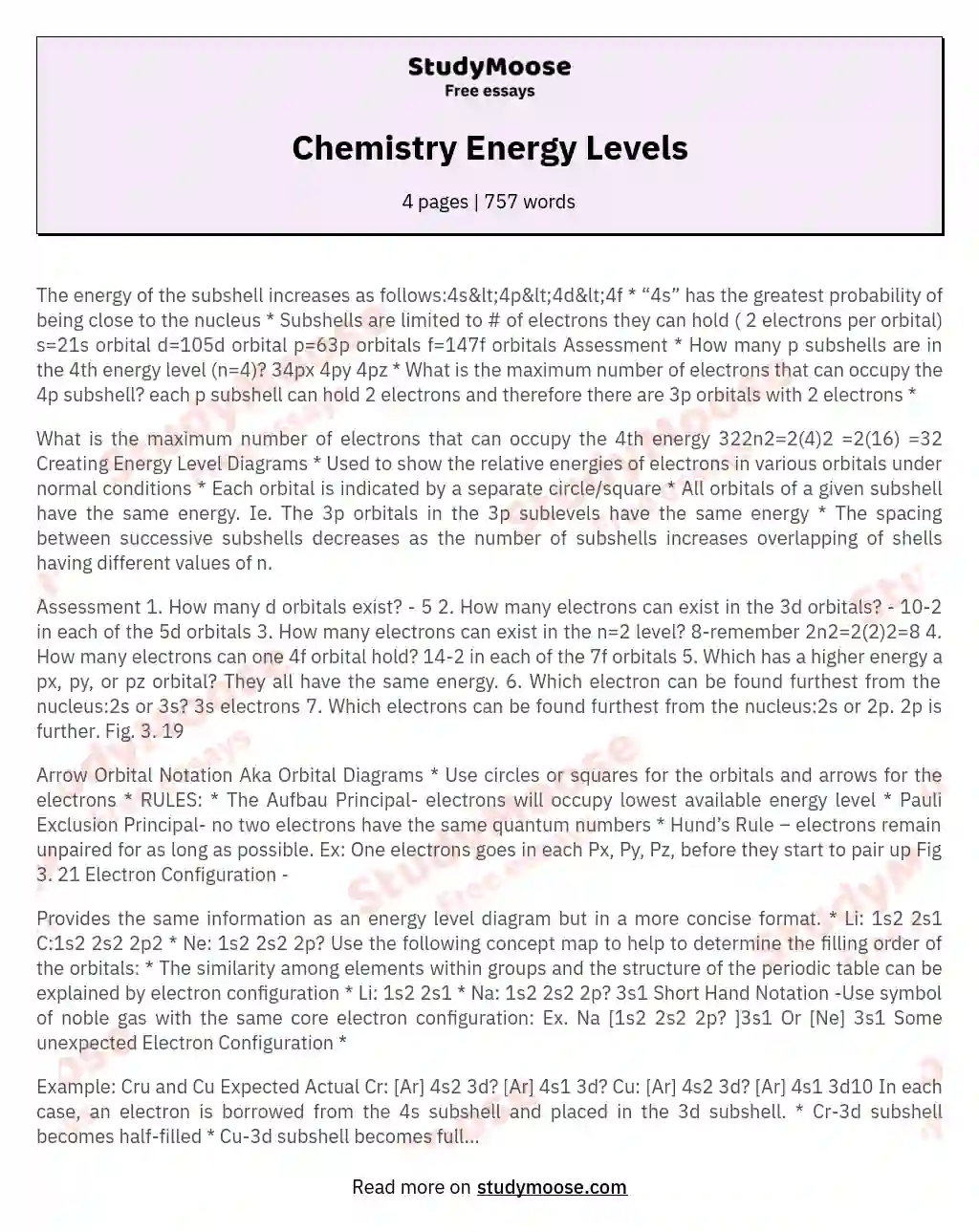Chemistry Energy Levels

Categories: Chemistry

The energy of the subshell increases as follows:4s&lt;4p&lt;4d&lt;4f * “4s” has the greatest probability of being close to the nucleus * Subshells are limited to # of electrons they can hold ( 2 electrons per orbital) s=21s orbital d=105d orbital p=63p orbitals f=147f orbitals Assessment * How many p subshells are in the 4th energy level (n=4)? 34px 4py 4pz * What is the maximum number of electrons that can occupy the 4p subshell? each p subshell can hold 2 electrons and therefore there are 3p orbitals with 2 electrons *

What is the maximum number of electrons that can occupy the 4th energy 322n2=2(4)2 =2(16) =32 Creating Energy Level Diagrams * Used to show the relative energies of electrons in various orbitals under normal conditions * Each orbital is indicated by a separate circle/square * All orbitals of a given subshell have the same energy.

Ie. The 3p orbitals in the 3p sublevels have the same energy * The spacing between successive subshells decreases as the number of subshells increases overlapping of shells having different values of n.

Get quality help nowDoctor JenniferVerified writer

Proficient in: Chemistry5 (893)

“ Thank you so much for accepting my assignment the night before it was due. I look forward to working with you moving forward ”+84 relevant experts are online

Assessment 1. How many d orbitals exist? – 5 2. How many electrons can exist in the 3d orbitals? – 10-2 in each of the 5d orbitals 3. How many electrons can exist in the n=2 level? 8-remember 2n2=2(2)2=8 4. How many electrons can one 4f orbital hold? 14-2 in each of the 7f orbitals 5. Which has a higher energy a px, py, or pz orbital? They all have the same energy. 6. Which electron can be found furthest from the nucleus:2s or 3s? 3s electrons 7.

Get to Know The Price Estimate For Your Paper
Topic
Number of pages
Email Invalid email

You won’t be charged yet!

Which electrons can be found furthest from the nucleus:2s or 2p. 2p is further. Fig. 3. 19

Arrow Orbital Notation Aka Orbital Diagrams * Use circles or squares for the orbitals and arrows for the electrons * RULES: * The Aufbau Principal- electrons will occupy lowest available energy level * Pauli Exclusion Principal- no two electrons have the same quantum numbers * Hund’s Rule – electrons remain unpaired for as long as possible. Ex: One electrons goes in each Px, Py, Pz, before they start to pair up Fig 3. 21 Electron Configuration –

Provides the same information as an energy level diagram but in a more concise format. * Li: 1s2 2s1 C:1s2 2s2 2p2 * Ne: 1s2 2s2 2p? Use the following concept map to help to determine the filling order of the orbitals: * The similarity among elements within groups and the structure of the periodic table can be explained by electron configuration * Li: 1s2 2s1 * Na: 1s2 2s2 2p? 3s1 Short Hand Notation -Use symbol of noble gas with the same core electron configuration: Ex. Na [1s2 2s2 2p? ]3s1 Or [Ne] 3s1 Some unexpected Electron Configuration *

Example: Cru and Cu Expected Actual Cr: [Ar] 4s2 3d? [Ar] 4s1 3d? Cu: [Ar] 4s2 3d? [Ar] 4s1 3d10 In each case, an electron is borrowed from the 4s subshell and placed in the 3d subshell. * Cr-3d subshell becomes half-filled * Cu-3d subshell becomes full * Half-filled and fully filled subshells tend to be more stable * Other expectations: Ag: [Kr] 4s2 3d10 Au: [Xe] 4f14 5d10 6s1 Explaining Ion Charges * Remember s electrons are lost before d electrons when dealing with transition metals. Ex1. Zn Zn: [Ar] 4s2 3d10 Zn2+: [Ar] 3d10 (4s electrons are lost so that the 3d orbital remains full) Ex2. Pb Pb: [Xe] 6s2 4f14 5d10 6p2 Pb2+: [Xe] 4f14 5d10 6p2 (The 6s electrons are lost)

Pb4+: [Xe] 4f14 5d10 (The 6p electrons are lost as well as the 6s electrons) Quantum Numbers * Electron waves (orbitals) can be characterized by a set quantum numbers, n, l, ml, ms Principle quantum number (n): * Identifies the energy of an electron in an orbital * All orbitals that have the same value of n are said to be in the same shell * Range from n=1 to n=infinity * Determines the size of the electron wave how far the wave extends from the nucleus * As n increases the energies of the orbitals also increase Secondary quantum number (l):

Divides the shells into smaller groups called subshells * n determines the values of l * for any given n, l may range from l=0 to l=n-1 * identifies the shape of the orbital Value of l| 0| 1| 2| 3| Letter designation| s(shape)| p(principle)| d(diffuse)| f(fundamental)| Magnetic quantum number (ml): * splits the subshells into individual orbits * identifies the orientation of the orbital * for any given value of l, ml has a value ranging from +l to –l * e. g. If l=0, ml=0; for l=1, ml=+1, 0, -1 which correspond to the x, y and z orientations of the p orbitals.